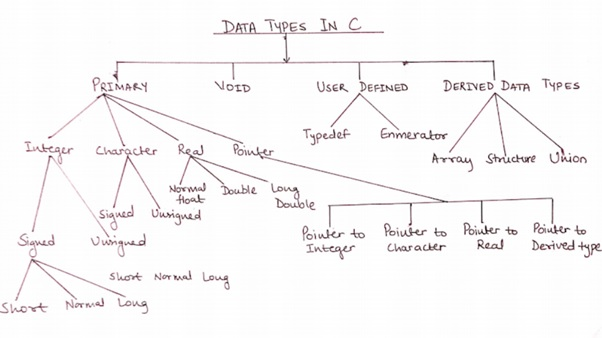# 9 Basic, Derived and User Defined Data Types in C Explained with Example

In this tutorial, we are learning different data types used in C programming along with the range of each data type.

### Introduction of Data and Datatype in C

What is Data in Programming?

It is generally known as useful information which is referred, stored and processed as per the processor’s requirements.

What is Data Type in Programming?

Now, this information is stored in various formats as per the nature of datum.

The C programming language provides us with a wide and vivid set of data types, such as int, float, char, etc.

## Different Data Types in C with their Ranges:

Data types in C are majorly categorized into 3 types.

• Basic Data Types in C
• User defined data types in C
• Derived Data type in CAs per the above schematic diagram, data types are bifurcated based on the nature of the data that gets stored.

Moreover, these data types differ in size too.

Generally, the signed, unsigned character and signed, unsigned short integer is of 8 bits. The signed and unsigned integer of 16 bits.

And signed, unsigned integer and float have a size of 32 bits.

For the need of greater sized data types, double(size:64 bits) and long double(size: 80 bits) are used.

### Primary / Basic Data Types in C

The basic datatypes are already defined in C programming, so you can use them  directory in your code.

#### 1. Integer:

‘int’ is the data type used to represent integer values of the decimal number system. It is further classified into signed and unsigned. The signed int is used for the integers on the left as well as the right of zero on the number line. Whereas unsigned int is used for the integers on the right of zero on the number line.

#### 2. Character:

This data type is used for the representation of characters. Its size is 8 bits and the range is different for the signed and unsigned one.

#### 3. Real:

This data type represents all the numbers whether decimal or integer, present on the number line. Depending upon the size it is classified into further three categories- float, double and long double.

#### 4. Pointer:

These are special data types that hold the address of the variable they point to. ‘&’ and ‘*’ are the two operators that are used when pointers are implemented. ‘&’ operator gives the address of the variable, whereas ‘*’ operator accesses the value at an address.

### User Defined Data Types in C

User has to define these data types before using it.

#### 5. Typedef:

Typedef, an abbreviation for type definition is a user-defined data type. Which means, it defines an identifier that can represent an existing data type. This data type increases the readability of codes with greater complexity.
Example:

```typedef int numbers;
numbers a=1;```

The above code states that numbers can be used to declare variables of type int.

#### 6. Enumerated:

It is another user-defined data type which does the job of creating a data type that can be assigned a value from a specific set of values.

It is declared by using the keyword ‘enum’.

Example:

```enum prime (2,3,5,7,11,13,17,19);
enum prime a,b;
a=5;
b=19;```

### Derived Data Types in C

These data types are derived from the basic data types.

#### 7. Array:

It’s the collection of homogeneous data types that are stored in contiguous memory cells and locations.

Example:

`int a`

It has 7 memory cells to store the 7 data elements as a, a, a, a, a, a and a.

Here, above integer array is derived from the basic data type ‘int’.

#### 8. Structure:

It’s the collection of non-homogeneous data types. It is very popular as it stores all the properties of an element.

Example:

```struct product
{
char name;
int pnum;
char consumer;
int cnum;
}```

Above example is the collection of basic data types like char and int.

#### 9. Union:

This data type is very similar to the structure as this one too stores non-homogeneous data types.

Example:

```union product
{
char name;
int pnum;
char consumer;
int cnum;
}```

What is the difference between structure and union in C?

They are different in terms of storage. In structure, each member has its own memory location, whereas all the members of a union share the same memory locations.

### Derived Data Types vs User Defined Data types in C

What is the difference between Derived data type and User Defined data types in C?

• Derived data types are created from basic data types such as int, float, char, etc.
• Example of Derived Data Types in C: Arrays, Pointers, Structures, etc.
• Using user-defined data types, the programmer can invent his/her own data types in C programming. It does not necessary to use basic data types to create user-defined data types in C.
• Example for User Defined Data Types in C: typedef (type definition), enum (enumerated data type)

### Data Value Ranges for Each Datatype in C

TYPE SIZE RANGE
Signed char 8 bits -128 to 127
Unsigned char 8 bits 0 to 255
Signed int 16 bits -32768 to 32767
Unsigned int 16 bits 0 to 65535
Signed short int 8 bits -128 to 127
Unsigned short int 8 bits 0 to 255
Signed long int 32 bits -2147483648 to 2147483647
Unsigned long int 32 bits 0 to 429967295
Float 32 bits 3.4E-38 to 3.4E+38
Double 64 bits 1.7E-308 to 1.7E+308
Long double 80 bits 3.4E-4932 to 1.1E+4932

In C and C++, Each data type has its storage classes in programming.

The detail about each of the data types we will see in the next part of this tutorial.

This is all about different data types used in C programming. If you have any specific questions to ask, write in the comment section below.

Happy Programming!